### Linear Integrated Circuits (LIC) - Unit 2 and 3 Some Important Two Marks

Anna University
Linear Integrated Circuits (LIC)
Unit 2 and 3 Some Important Two Marks

```
```

`1. Slew rate:`

```The slew rate is defined as the maximum rate of change of output voltage caused by a step input
voltage. It is given by incapacitor.

CMRR:

The ratio of differential gain and the common mode gain is termed as Common Mode Rejection Ratio.

2. Circuit diagram of differentiator using op-amp.```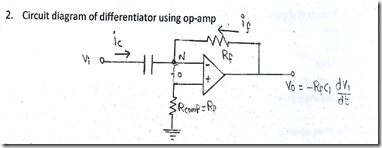3. Why op-amp configurations are not used in linear applications?

An op-amp in open loop configuration will not be used for linear applications because the output of OP-AMP in open loop mode will be ± V sat.

4. For an inverting summer find Vo in terms of two input Vi & V2.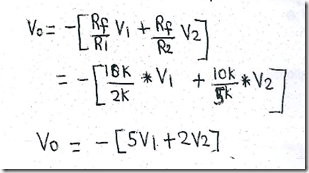5. What is the need for compensating network in op-amp?

In order to attain

> Larger bandwidth

> Lower closed loop gain

> Single break frequency

> Central the phase shift and stability

6. How many resistors are required in a 10-bit weighted resister D/A convertor?
10(ten) resistors are required in a 10-bit weighted resistor D/A convertor.

7. What is the advantage of active op-amp based clipper over passive clipper?

The advantage of active op-amp based clipper over passive clipper is as follows,

> Flexible design

> Accuracy

> Less noise components

> Isolation between input circuits and load circuits.

8. List any four important applications of a comparator.

> Zero crossing detector

. > Window detector

> Time marker generator

> Phase meter

9. State the two advantages of active filters over passive filters.

> Flexible design

> Accuracy

> Less noise components

> Isolation between input circuits and load circuits.

10. What are the basic requirements of a good instrumentation amplifier?

> High gain

> Accuracy

> High CMRR

> Low DC offset

> Low input impedance

11 The two input bias current of an op-amp are 22pA & 26mA What is the value of input offset current and input bias current?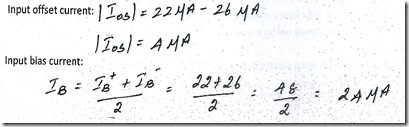12 Sketch the circuit of an op amp employed as, non inverting zero crossing detector, along with input and output waveform.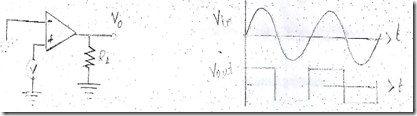13 An op amp data sheet gives 15V power bandwidth of 25KHZ What is the slew rate?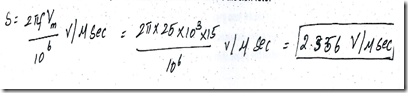14. Compare ideal and practical characteristics of an operational amplifier.

An ideal op amp draws no current from the source and its response is also independent of temperature. However a reach op amp does not work this way Current is taken from the source into the op amp inputs Also the two input respond differently to current and voltage due to mismatch in transistor.

15 Draw the circuit diagram of op-amp integrator Mention its applications.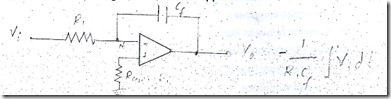Applications

> The gain of an integrator at low frequency can be limited to avoid the saturation problem.

16. Draw the circuit of current to voltage convertor using op-amp and bring out the relation. State the application.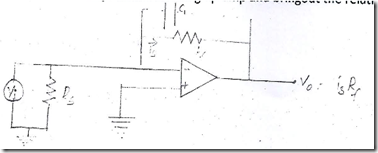Application:

> The photocell, photodiode and photovoltaic cell gives an output current that ¡s proportional to an incident radiant energy or light.

> The current through these devices can be converted into voltage by using an I to V converter and thereby the amount of light or radiant energy incident on the photo device can be measured.

17. Which is fastest ADC and why?

Successive approximation type.

i. It is faster(tracking circuit) .

ii. The successive approximation type uses very efficient code search strategy to complete n-bit conversion in just n-clock periods.

18. How many resistors are required in a 10-bit weighted resister D/A convertor?

10(ten) resistors are required in a 10-bit weighted resistor D/A convertor.

19. A 8 bit DAC has resolution of 2OmV/bit. what is the analog output voltage for the digital input code 00010110 (the MSB is the left most bit)?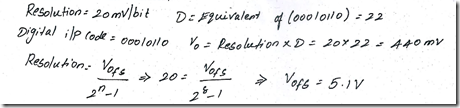20. What is zero crossing detector?

It is provided that Vref is set to zero. It detects how many time cross the zero value of electrical quantity.

#### 1 comment:

1.You made such an interesting piece to read, giving every subject enlightenment for us to gain knowledge. Thanks for sharing the such information with us to read this... electronic component